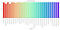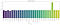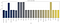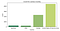# Hands-On Time-Series Forecasting in Python

## “The goal of forecasting is not to predict the future but to tell you what you need to know to take meaningful action in the present” ~ Paul Saffo

Time Series forecasting is an important concept in Statistics as well as data science. In this article, I’ll be covering an in-depth hands-on exercise for Time Series Analysis.

Before starting with the Python code, let’s discuss some very basic points of Time Series Analysis. For those who are new to it, I’ve attached some links in references to get the complete overview of Time Series Analysis.

As we know, statistical data is of 3 types:

1. Time series data: A set of observations on the values that a variable takes at different times.
2. Cross-sectional data: Data of one or more variables, collected at the same point in time.
3. Pooled data: A combination of time series data and cross-sectional data.

Thus, the study of one variable for a period of time is called time series analysis.

The objective of time series analysis is to understand the process which is generating the series and forecast future values of a variable under study.

There are 4 components of a Time Series:

1. Trend (T): This is the general, long term tendency of the data to increase or decrease with time.
2. Seasonal (S): It is the non-random component that repeats itself at regular intervals.
3. Cyclic [C]: Oscillatory movements with a period of more than 1 year are cyclic variations.
4. Random (R): Any movement of Time Series that can’t be accounted for by the above 3 components, is accounted in random variation. These are unforeseen events, famines etc.

We always decompose a Time Series into different components as we might be interested in a particular component.

## Mathematical Model of Time Series

1. Multiplicative: It is given by ->T*S*C*R

Once, we decompose a Time series, based on the type of mathematical model, we can separate various components. Later in this article, I’ll show how to do this using Python.

ARIMA and ARMA are 2 common models used in the analysis and forecasting of Time Series data.

# ARMA

In the AR model, the current value is expressed as a linear function of a finite number of previous values and a random shock.

In the MA model, the current value is expressed as a linear function of the current and finite number of previous shocks.

Thus, ARMA is a combination of both of these.

To get the value of p (lag order), we use PACF (Partial Autocorrelation Function) and for q (order of Moving average), we use ACF (Autocorrelation Function).

# ARIMA

This model has both AR and MA, along with ‘I’ part. Here, the ‘integrated’ part means differencing. Its order is denoted by d and is called ‘Order of Differencing’. Differencing is done to make a series stationary.

# Now, let’s start to code.

(P.S.:This is not a promotional post or comment. Anyone can join this community free of cost, interact with members and do these projects. So, if you’re looking to do projects and learn from others, give it a try!)

`Description of dataset:This dataset shows traffic to and from the Airport as a Percentage of the Traffic volume during the baseline period. The baseline period used for computing this metric is from 1st Feb to 15th March 2020.`

Importing necessary libraries

`import pandas as pdimport numpy as npimport seaborn as snsimport matplotlib.pyplot as pltimport warningswarnings.filterwarnings("ignore")`

Getting Data

`data = pd.read_excel('F:\Cheenta\Project1\covid_impact_on_airport_traffic.xlsx')data.head(2)`

Knowing data

`data.tail(2)`
`data.describe(include='all')`

There are 4 countries, 23 states,27 cities and 28 airports in the dataset. Data has been collected for 262 distinct days starting from 16/03/20 to 02/12/20.

Centroid and Country are POINT and POLYGON structures, telling us that they are geographical locations.

Also, from the count, we can see, that all features have no missing value.

## Removing features that aren’t important

The same reasoning applies to the ‘centroid’. Centroid probably tells us the latitude and longitude of the centre of the airport. Since we don’t have any use for that. We’ll drop it too.

ISO_3166_2 is some unique value for every state. We won’t be requiring it for time series analysis.

AggregationMethod is always ‘Daily’, so, it doesn’t provide any information. We can remove it.

No information on the version is provided. So, we’ll leave that from our analysis.

`data.head()`

Univariate Analysis

`plt.figure(figsize=(20,6))fig1 = sns.countplot(x = 'AirportName', data = data , palette='rainbow_r')fig1.set_xticklabels(fig1.get_xticklabels(), rotation=90)fig1.set_title("Count for various Airports")plt.show()`This shows that there are around 250 data points on each airport except Santiago International Airport and Edmonton International.
`plt.figure(figsize=(20,6))fig2 = sns.countplot(x = 'City', data = data , palette='viridis')fig2.set_xticklabels(fig2.get_xticklabels(), rotation=90)fig2.set_title("Count for various City")plt.show()`This shows that all cities have more or less equal counts in data except New York. The most likely reason would be that it has more airports.
`plt.figure(figsize=(20,6))fig3 = sns.countplot(x = 'State', data = data , palette='cividis')fig3.set_xticklabels(fig3.get_xticklabels(), rotation=90)fig3.set_title("Count for various State")plt.show()`Here, all states have equal counts in data except Alberta, Quebec, California and New York. Again most likely reason must be the number of airports. We’ll come again on this in an in-depth analysis of countries.
`plt.figure(figsize=(8,4))fig4 = sns.countplot(x = 'Country', data = data , palette='summer')fig4.set_xticklabels(fig4.get_xticklabels())fig4.set_title("Count for various Country")plt.show()`Maximum data points are for the US followed by Canada. This is because the number of airports in the US and Canada is probably more than in Australia and Chile.

Checking our inferences of Univariate plots

`data.groupby("Country")['State','City','AirportName'].nunique()`

## Bivariate Analysis

`fig5 = sns.pairplot(data,hue='Country',height=5,palette='husl',aspect=1)fig5._legend.remove()plt.title("Distribution of Percent of Baseline for different countries")plt.legend(loc = 'upper right',bbox_to_anchor=(1.2, 0., 0.5, 0.5))plt.show()`

# Analysis for Chile

`# Data on Chile is based on only one state which has one city with one airport named Santiago International airport.data_chile = data_chile.sort_values(by="Date")data_chile.set_index('Date',inplace=True)data_chile = data_chile.drop(columns=['AirportName','City','State','Country'])data_chile.head()`
`plt.figure(figsize=(20,10))plt.plot(data_chile['PercentOfBaseline'])plt.title("Plot for PercentOfBaseline Vs Time for Chile")plt.show()`
`from statsmodels.tsa.stattools import adfullerprint('Results of Dickey-Fuller Test:')dftest = adfuller(data_chile['PercentOfBaseline'], autolag='AIC')dfoutput = pd.Series(dftest[0:4], index=['Test Statistic','p-value','#Lags Used','Number of Observations Used'])for key,value in dftest.items():    dfoutput['Critical Value (%s)'%key] = valueprint(dfoutput)`

Dickey-Fuller Test: This is one of the statistical tests for checking stationarity. Here the null hypothesis is that the TS is non-stationary. If the ‘Test Statistic’ is less than the ‘Critical Value’, we can reject the null hypothesis and say that the series is stationary.

We can conclude that our data is not stationary, hence, we need to make it stationary because all time-series models are stationary.

`#define function for kpss testfrom statsmodels.tsa.stattools import kpss#define KPSSprint ('Results of KPSS Test:')kpsstest = kpss(data_chile['PercentOfBaseline'], regression='c')kpss_output = pd.Series(kpsstest[0:3], index=['Test Statistic','p-value','Lags Used'])for key,value in kpsstest.items():    kpss_output['Critical Value (%s)'%key] = valueprint (kpss_output)`

The authors of the KPSS test have defined the null hypothesis as the process is trend stationery, to an alternate hypothesis of a unit root series. KPSS test also suggests that our series is NOT stationary.

`data_chile['diff'] = data_chile['PercentOfBaseline'] - data_chile['PercentOfBaseline'].shift(1)plt.figure(figsize=(20,10))plt.plot(data_chile['diff'])plt.title("Plot for lagged PercentOfBaseline Vs Time for Chile")plt.show()`
`from statsmodels.tsa.seasonal import seasonal_decomposedecomposition = seasonal_decompose(x=data_chile['PercentOfBaseline'].dropna(),model='multiplicative',freq=9)trend = decomposition.trendseasonal = decomposition.seasonalresidual = decomposition.residplt.figure(figsize=(10,10))plt.subplot(411)plt.plot(data_chile['PercentOfBaseline'], label='Original')plt.legend(loc='best')plt.subplot(412)plt.plot(trend, label='Trend')plt.legend(loc='best')plt.subplot(413)plt.plot(seasonal,label='Seasonality')plt.legend(loc='best')plt.subplot(414)plt.plot(residual, label='Residuals')plt.legend(loc='best')plt.tight_layout()`
`from statsmodels.graphics.tsaplots import plot_acf,plot_pacfplot_acf(data_chile['diff'].dropna(),zero=False)plt.xlim(0,20)plt.xticks(np.arange(0,20,1))plt.show() #q=1 or q=0`

Value of q is determined by taking the 1st significant lag after which acf value falls in the limits (blue shading) or become insignificant. Here, q=1.

`plot_pacf(data_chile['diff'].dropna(),zero=False,lags=40,method='ols',alpha=0.05)plt.xticks(np.arange(0,40,2))plt.show() # p=3,5`

Similarly, the Value of p comes out to be 3.

`df = pd.DataFrame(data_chile['diff'])df.dropna(inplace=True)from statsmodels.tsa.arima_model import ARMA# Create Training and Testtrain = df.iloc[:212]test = df.iloc[212:]# Build Modelmodel = ARMA(train, order=(6,0))  fitted = model.fit()  print(fitted.summary())# Forecastfc, se, conf = fitted.forecast(25, alpha=0.05)  # 95% conf# Make as pandas seriesfc_series = pd.Series(fc, index=test.index)lower_series = pd.Series(conf[:, 0], index=test.index)upper_series = pd.Series(conf[:, 1], index=test.index)# Plotplt.figure(figsize=(12,5), dpi=100)plt.plot(train, label='training')plt.plot(test, label='actual',color='r')plt.plot(fc_series, label='forecast',color='g')plt.fill_between(lower_series.index, lower_series, upper_series,color='g', alpha=.05)plt.title('Forecast vs Actuals')plt.legend(loc='best', fontsize=8)plt.show()`

The value of p and q give us an idea. We have to run various models close to those values and take the best model. The best Model is the one that has the lowest AIC, highest log-likelihood and the coefficients of the model must be significant.

Tip: You can get the same model using ARIMA(6,1,0) on Chile’s PercentOf Baseline. Since I have previously differenced the series, I didn’t use ARIMA here.

# Analysis for the USA

`data_US = data[data['Country']=='United States of America (the)']df1 = pd.DataFrame(data_US.groupby('Date',as_index=True)['PercentOfBaseline'].mean())df1.head()`

Here, we will take mean of all because, in the USA, we have 16 airports. So, we’ll take the mean of all values and model the data.

`plt.figure(figsize=(20,10))plt.plot(df1)plt.title("Plot of USA's average PercentOfBaseline Vs Time")plt.show()`

This series is stationary with time according to the ADF test. So, we’ll model it directly and no need for differencing.

The model will be best represented by ARMA(1,2).

The graph looks like this:

I’ve shown the main steps in the analysis in sequential order. To see all the steps of the analysis, please see my repository at this link.

Also, for practice, you can do a similar analysis for Australia and Canada.

Hope you liked the article. Feel free to post comments.

For more such articles on data science, follow me on medium.

## Nerd For Tech

From Confusion to Clarification

## Nerd For Tech

NFT is an Educational Media House. Our mission is to bring the invaluable knowledge and experiences of experts from all over the world to the novice. To know more about us, visit https://www.nerdfortech.org/.

Written by

## Anjali Pal

A data science enthusiast who believes that “It is a capital mistake to theorize before one has data”- Sherlock Holmes. Visit me at https://anjali001.github.io## Nerd For Tech

NFT is an Educational Media House. Our mission is to bring the invaluable knowledge and experiences of experts from all over the world to the novice. To know more about us, visit https://www.nerdfortech.org/.